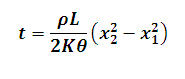# Time for Accretion of Ice Calculator

This Calctown Calculator calculates the time of accretion of a layer of ice.

kg/m3
J/kg
W/(m.K)
K
m
m

#### Result

swhere

t = time for accretion

ρ = density of fluid

K = thermal conductivity

θ = temperature between water below and air above the ice layer

x1 = initial thickness of ice layer

x2 = final thickness of ice layer